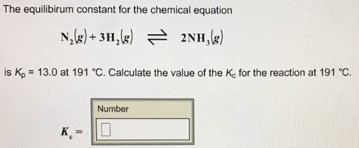# Problem: The equilibrium constant for the chemical equation N2(g) + 3H2(g) ⇌ 2NH3(g) is Kp = 13.0 at 191°C. Calculate the value of the Kc for the reaction at 191°C. Kc =

###### FREE Expert Solution
88% (10 ratings)###### Problem Details

The equilibrium constant for the chemical equation

N2(g) + 3H2(g) ⇌ 2NH3(g)

is Kp = 13.0 at 191°C. Calculate the value of the Kc for the reaction at 191°C.

Kc =